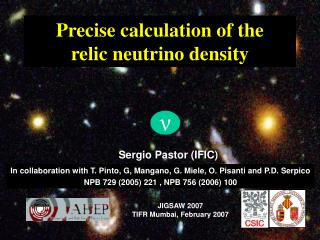DownloadDownload PresentationPrecise calculation of the relic neutrino density

# Precise calculation of the relic neutrino density

Download Presentation## Precise calculation of the relic neutrino density

- - - - - - - - - - - - - - - - - - - - - - - - - - - E N D - - - - - - - - - - - - - - - - - - - - - - - - - - -
##### Presentation Transcript

1. ν Precise calculation of the relic neutrino density Sergio Pastor (IFIC) In collaboration with T. Pinto, G, Mangano, G. Miele, O. Pisanti and P.D. Serpico NPB 729 (2005) 221 , NPB 756 (2006) 100 JIGSAW 2007 TIFR Mumbai, February 2007

2. Introduction: the Cosmic Neutrino Background Relic neutrino decoupling New results in the SM and in presence of electron-neutrino NSI Outline Precise calculation of the relic neutrino density

3. The CNB

4. Neutrinos coupled by weak interactions(in equilibrium) T~MeV t~sec Primordial Nucleosynthesis

5. Neutrinos coupled by weak interactions(in equilibrium) Free-streaming neutrinos (decoupled) Cosmic Neutrino Background Neutrinos keep the energy spectrum of a relativistic fermion with eq form Primordial Nucleosynthesis T~MeV t~sec

6. Neutrinos decoupled at T~MeV, keeping a spectrum as that of a relativistic species The Cosmic Neutrino Background • Number density • Energy density Massless Massive mν>>T

7. Relic neutrino decoupling

8. Neutrinos in Equilibrium 1 MeV  T mμ Tν= Te = Tγ

9. Neutrino decoupling As the Universe expands, particle densities are diluted and temperatures fall. Weak interactions become ineffective to keep neutrinos in good thermal contact with the e.m. plasma Rough, but quite accurate estimate of the decoupling temperature Rate of weak processes ~ Hubble expansion rate Since νe have both CC and NC interactions withe± Tdec(νe) ~ 2 MeV Tdec(νμ,τ) ~ 3 MeV

10. Neutrinos coupled by weak interactions(in equilibrium) Free-streaming neutrinos (decoupled) Cosmic Neutrino Background Neutrinos keep the energy spectrum of a relativistic fermion with eq form T~MeV t~sec

11. Neutrino and Photon (CMB) temperatures At T~me, electron-positron pairs annihilate heating photons but not the decoupled neutrinos

12. Precise calculation of neutrino decoupling: SM + flavour oscillations

13. Non-instantaneous neutrino decoupling At T~me, e+e- pairs annihilate heating photons But, since Tdec(ν) is close to me, neutrinos share a small part of the entropy release f=fFD(p,T)[1+δf(p)]

14. Neutrino and Photon (CMB) temperatures At T~me, electron-positron pairs annihilate heating photons but not the decoupled neutrinos

15. + evolution of total energy density: Momentum-dependent Boltzmann equation Statistical Factor 9-dim Phase Space Pi conservation Process

16. Evolution of fν for a particular momentum p=10T At lower temperatures distortions freeze out Between 2>T/MeV>0.1 distortions grow For T>2 MeV neutrinos are coupled

17. Final spectral distortion Evolution of fν for a particular momentum p=10T

18. δf x10 e ,

19. Relativistic particles in the Universe At T<me, the radiation content of the Universe is

20. # of flavour neutrinos: Relativistic particles in the Universe At T<me, the radiation content of the Universe is Effective number of relativistic neutrino species Traditional parametrization of the energy density stored in relativistic particles Bounds from BBN and from CMB+LSS

21. # of flavour neutrinos: Relativistic particles in the Universe At T<me, the radiation content of the Universe is Effective number of relativistic neutrino species Traditional parametrization of the energy density stored in relativistic particles Neff is not exactly 3 for standard neutrinos

22. Results Dolgov, Hansen & Semikoz, NPB 503 (1997) 426 Mangano et al, PLB 534 (2002) 8

23. Neutrino oscillations in the Early Universe Neutrino oscillations are effective when medium effects get small enough Compare oscillation term with effective potentials Coupled neutrinos Oscillation term prop. to Δm2/2E Second order matter effects prop. to GFE/MZ2[ρ(e-)+ρ(e+)] First order matter effects prop. to GF[n(e-)-n(e+)] Strumia & Vissani, hep-ph/0606054 Previous work by Hannestad, PRD 65 (2002) 083006

24. Effects of flavour neutrino oscillations on the spectral distortions The variation is larger for e Around T~1 MeV the oscillations start to modify the distortion

25. Effects of flavour neutrino oscillations on the spectral distortions The variation is larger for e Around T~1 MeV the oscillations start to modify the distortion The difference between different flavors is reduced Oscillations smooth the flavour dependence of the distortion

26. Results Mangano et al, NPB 729 (2005) 221

27. Contribution of neutrinos to total energy density today (3 degenerate masses) Present neutrino number density Changes in CNB quantities

28. Precise calculation of neutrino decoupling: Non-standard neutrino-electron interactions

29. New effective interactions between electron and neutrinos Electron-Neutrino NSI

30. Limits on from scattering experiments, LEP data, solar vs Kamland data… Electron-Neutrino NSI Breaking of Lepton universality (=) Flavour-changing (≠ ) Berezhiani & Rossi, PLB 535 (2002) 207 Davidson et al, JHEP 03 (2003) 011 Barranco et al, PRD 73 (2006) 113001

31. Analytical calculation of Tdec in presence of NSI SM SM Contours of equal Tdec in MeV with diagonal NSI parameters

32. Neff varying the neutrino decoupling temperature

33. Effects of NSI on the neutrino spectral distortions Here larger variation for , Neutrinos keep thermal contact with e- until smaller temperatures

34. Results Very large NSI parameters, FAR from allowed regions Mangano et al, NPB 756 (2006) 100

35. Results Large NSI parameters, still allowed by present lab data Mangano et al, NPB 756 (2006) 100

36. Departure from Neff=3 not observable from present cosmological data Mangano et al, hep-ph/0612150

37. …but maybe in the near future ? Forecast analysis: CMB data ΔNeff ~ 3 (WMAP) ΔNeff ~ 0.2 (Planck) Bowen et al MNRAS 2002 Example of future CMB satellite Bashinsky & Seljak PRD 69 (2004) 083002

38. Conclusions Cosmological observables can be used to bound (or measure) neutrino properties, once the relic neutrino spectrum is known ν The small spectral distortions from relic neutrino—electron processes can be precisely calculated, leading to Neff=3.046 (or up to 3 times more including NSI)